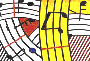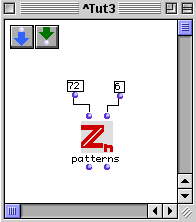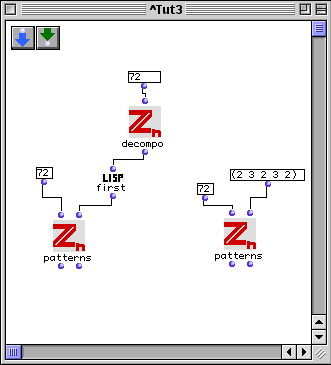Tutorial 3: Rhythmic patterns associated with a given period.We know from the 'decompo' function that the period n=72 can be decomposed in the five numbers:

p1=3

p2=2

n1=3

n2=2

n3=2

The Regular Complementary Canon of Maximal Category that is 'canonically' associated with this decomposition will have a number of voices equal to the product n1n2, i.e. 6.

The left evaluation gives the rhythmic pattern R that generates the Canon by the temporal translation specified by the pattern S that is given by the right output evaluation.You can also give the five numbers directly to the patterns function.